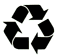# Online Math Notation for Calculus

It is difficult to write mathematical equations online. They won't look like the expressions you will see in textbooks or lectures. But, there are still ways to make yourself understood.

There are a few peculiarities about the way math has to be written online. Besides the familiar + and -, there are a few symbols that may be unfamiliar:
 symbol operation example / division x/2 * multiplication x*y ^ power (exponent) x^3 sqrt square root sqrt(x) exp exponential function exp(x)  or  e^x
For example, x-squared would be written as x^2. The expression:would be written sqrt(1-b*x^2) or √(1-b*x^2).

Einstein's famous formulawould be E=mc^2.

It is important that you use parentheses to make clear the order of operations. For example, 2/x+1 is not the same as 2/(x+1). The first expression says: divide two by x, then add one. The second expression says: divide 2 by the quantity x+1.

The order of operations is
^ and sqrt
* and /
+ and - .

Large numbers are written in scientific notation, using powers of 10. For instance, 100 is written as 102 or 1E+2, one billion is written as 109 or 1E+9. Such notation is also useful in describing things such as the National Debt  ;-)

Limits are written as follows:
lim(x-->0) [ f(x)]
Write infinity as oo: two lowercase 'O' in a smaller font:
lim(x-->oo) [1/x] = 0

Derivatives can be written as quotients: dy/dx. Integrals present more of a problem. I suggest
S f(x) dx
for the indefinite integral of the function f(x) over x. The limits for definite integrals need to be spelled out explicitly.This web page is made using recycled materials.
No electrons were destroyed (or created).

Back to
Calculus I | Calculus II